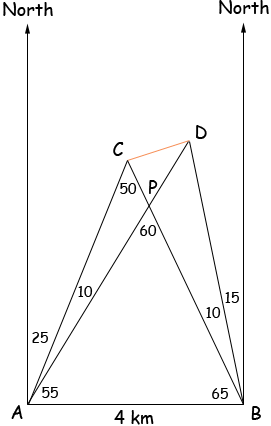Name: Greg Who is asking: Student Level of the question: Secondary Question: A and B are two towers, B being 4 km due east of A. The true bearings of a flagpole, C, from A and B are α east of north and α west of north respectively. The true bearings of a second flagpole, D, from A and B are (α + β) east of north and (α - β) west of north respectively. Assuming A, B, C, and D are on level ground, and that α = 25, β = 10, find the distance between C and D. Hi Greg, I drew a diagram to get you started. (The small numbers re angle measurements in degrees.)Using the law of sines and the triangle ABC find |BC|. Using the law of sines and the triangle ABP find |BP|. |PC| = |BC| - |BP|. Can you see how to proceed from here? Penny